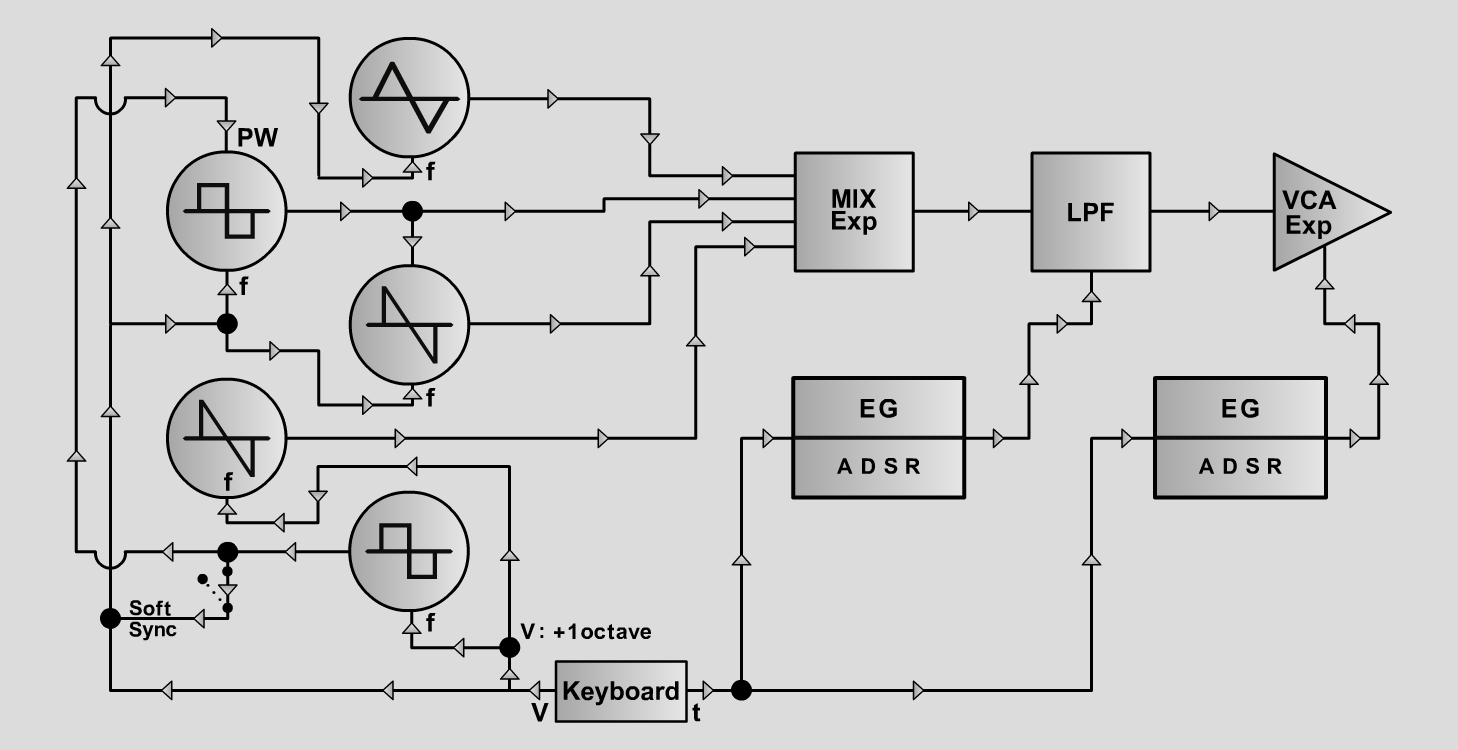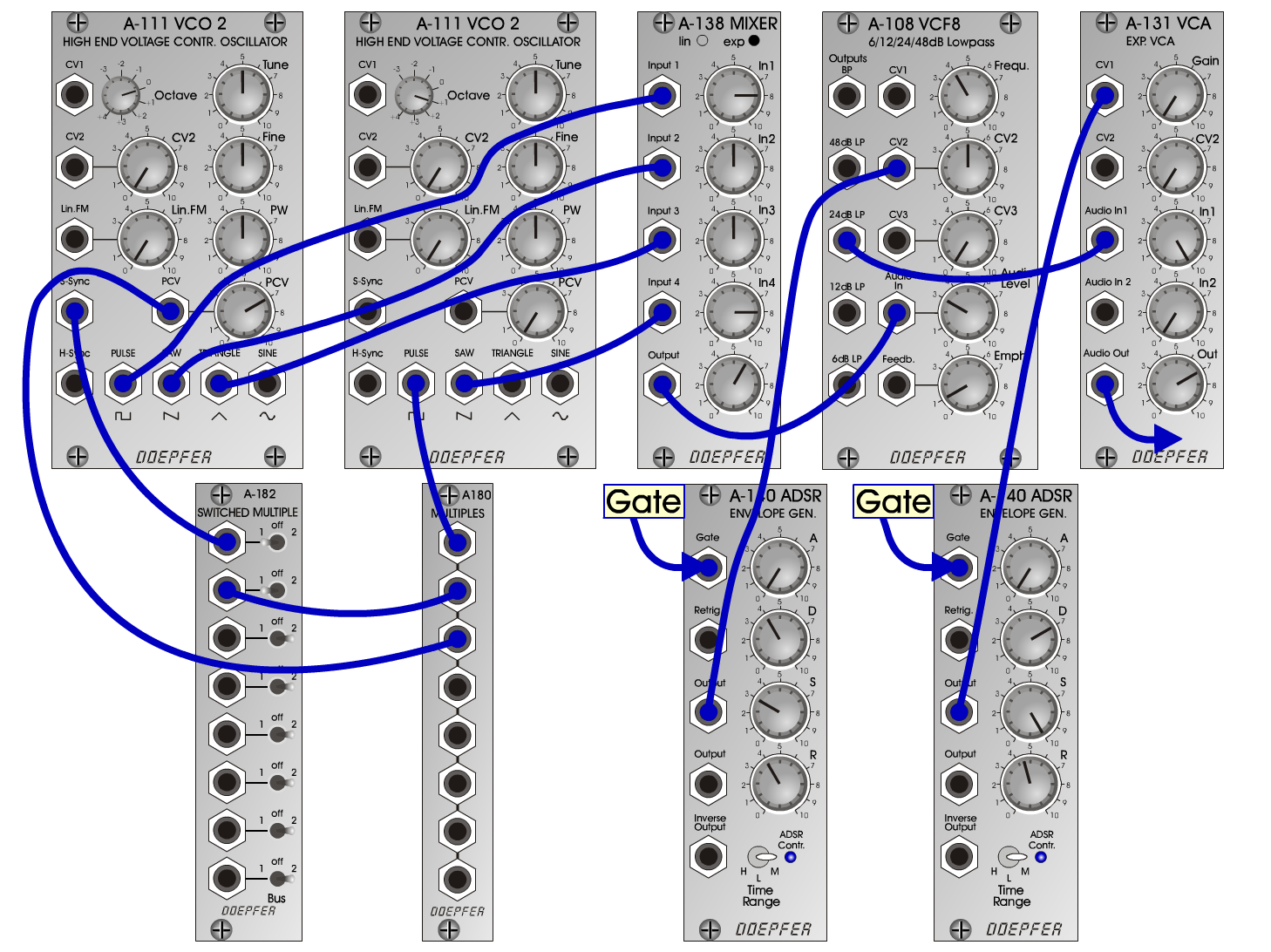Multiwave-LeadS y n t hD o e p f e r A - 100 Connections: Settings: Multiwave-Lead1 A-111/1 (Pulse) <=> A-138exp (Input 1)  A-111/1 (Saw) <=> A-138exp (Input 2)  A-111/1 (Tri) <=> A-138exp (Input 3)  A-111/2 (Saw) <=> A-138exp (Input 4)  A-111/2 (Pulse) <=> A-180  A-180 <=> A-182 (a)  A-182 (b) <=> A-111/1 (S-Sync)  A-180 <=> A-111/1 (PCV)  A-138exp (Output) <=> A-108 (Audio In)  A-108 (24 dB LP) <=> A-131 (Audio In)  A-140/1 (Output) <=> A-131 (CV1)  A-140/2 (Output) <=> A-108 (CV2) A-111/1 (Octave = 0, Tune = 5, PW = 5, PCV = 7)  A-111/2 (Octave = +1, Tune = 5, PW = 5)  A-138 (In 1 = 8, In 2 = 5, In 3 = 5, In 4 = 8, Out = 6)  A-108 (Frequ. = 4, CV2 = 5, Audio Level = 3, Emph. = 1)  A-131 (Gain = 0, Audio In 1 = 10, Audio Out = 7)  A-140/1 (A = 0, D = 7, S = 10, R = 4.5, Range = M)  A-140/2 (A = 0, D = 4, S = 3, R = 4, Range = M)  A-182 (a = 1, b = 1) Multiwave-Lead2 A-111/1 (Octave = 0, Tune = +10 cents, PW = 5, PCV = 7)  A-111/2 (Octave = +1, Tune = -10 cents, PW = 8)  A-138 (In 1 = 8, In 2 = 5, In 3 = 0, In 4 = 8, Out = 6)  A-108 (Frequ. = 4, CV2 = 5, Audio Level = 3, Emph. = 1)  A-131 (Gain = 0, Audio In 1 = 10, Audio Out = 7)  A-140/1 (A = 0, D = 7, S = 0, R = 4, Range = M)  A-140/2 (A = 0, D = 4, S = 3, R = 3, Range = M)  A-182 (a = 1, b = off) Multiwave-Lead3 A-111/1 (Octave = 0, Tune = +10 cents, PW = 5, PCV = 7)  A-111/2 (Octave = +1, Tune = -10 cents, PW = 8)  A-138 (In 1 = 8, In 2 = 5, In 3 = 0, In 4 = 8, Out = 6)  A-108 (Frequ. = 4, CV2 = 5, Audio Level = 3, Emph. = 1)  A-131 (Gain = 0, Audio In 1 = 10, Audio Out = 7)  A-140/1 (A = 2.5, D = 7, S = 0, R = 4, Range = M)  A-140/2 (A = 1, D = 4, S = 3, R = 3, Range = M)  A-182 (a = 1, b = off) Multiwave-Lead4 A-111/1 (Octave = 0, Tune = 5, PW = 5, PCV = 7)  A-111/2 (Octave = +1, Tune = 5, PW = 4)  A-138 (In 1 = 7, In 2 = 10, In 3 = 3, In 4 = 10, Out = 6)  A-108 (Frequ. = 4, CV2 = 4, Audio Level = 3, Emph. = 1)  A-131 (Gain = 0, Audio In 1 = 10, Audio Out = 7)  A-140/1 (A = 0, D = 6, S = 0, R = 4, Range = M)  A-140/2 (A = 0, D = 4, S = 3, R = 3, Range = M)  A-182 (a = 1, b = 1) Multiwave-Lead5 A-111/1 (Octave = 0, Tune = 5, PW = 5, PCV = 7)  A-111/2 (Octave = +1, Tune = 5, PW = 4)  A-138 (In 1 = 7, In 2 = 10, In 3 = 3, In 4 = 10, Out = 6)  A-108 (Frequ. = 4, CV2 = 4, Audio Level = 3, Emph. = 1)  A-131 (Gain = 0, Audio In 1 = 10, Audio Out = 7)  A-140/1 (A = 2, D = 6, S = 0, R = 4, Range = M)  A-140/2 (A = 3, D = 4, S = 3, R = 3, Range = M)  A-182 (a = 1, b = 1) Josef MuellerSound samples Bach Inventio 8 (BWV 779) Multiwave-Lead 1 A Multiwave-Lead 1 B Multiwave-Lead 1 C Multiwave-Lead 2 A Multiwave-Lead 2 B Multiwave-Lead 2 C Multiwave-Lead 3 A Multiwave-Lead 3 B Multiwave-Lead 3 C Multiwave-Lead 4 A Multiwave-Lead 4 B Multiwave-Lead 4 C Multiwave-Lead 5 A Multiwave-Lead 5 B Multiwave-Lead 5 C